# How to generate random numbers in Excel: RAND and RANDBETWEEN functions

The tutorial explains the specificities of the Excel random number generator algorithm and demonstrates how to use RAND and RANDBETWEEN functions to generate random numbers, dates, passwords and other text strings in Excel.

Before we delve into different techniques of generating random numbers in Excel, let's define what they actually are. In plain English, random data is a series of numbers, letters or other symbols that lacks any pattern.

Randomness has a variety of different applications in cryptography, statistics, lottery, gambling, and many other fields. And because it has always been in demand, various methods of creating random numbers have existed since ancient times, such as flipping coins, rolling dice, shuffling playing cards, and so on. Of course, we won't rely on such "exotic" techniques in this tutorial and focus on what Excel random number generator has to offer.

## Excel random number generator - the basics

Although the Excel random generator passes all standard tests of randomness, it does not generate true random numbers. But don't write it off immediately :) Pseudo-random numbers produced by the Excel random functions are fine for many purposes.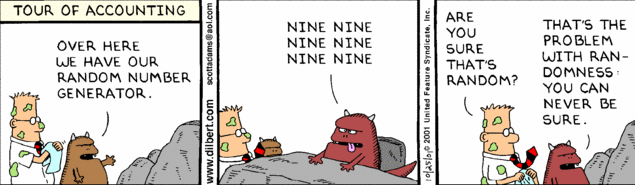Let's take a closer look at the Excel random generator algorithm so that you know what you can expect from it, and what you cannot.

Like most computer programs, Excel random number generator produces pseudo-random numbers by using some mathematical formulas. What it means for you is that, in theory, random numbers generated by Excel are predictable, provided that someone knows all the details of the generator's algorithm. This is the reason why it has never been documented and will hardly ever be. Well, what do we know about the random number generator in Excel?

• The Excel RAND and RANDBETWEEN functions generate pseudo-random numbers from the Uniform distribution, aka rectangular distribution, where there is equal probability for all values that a random variable can take on. A good example of the uniform distribution is tossing a single die. The outcome of the toss is six possible values (1, 2, 3, 4, 5, 6) and each of these values is equally likely to occur. For a more scientific explanation, please check out wolfram.com.
• There is no way to seed either Excel RAND or RANDBETWEEN function, which are rumored to be initialized from the computer's system time. Technically, a seed is the starting point for generating a sequence of random numbers. And every time an Excel random function is called, a new seed is used that returns a unique random sequence. In other words, when using the random number generator in Excel, you cannot get a repeatable sequence with the RAND or RANDBETWEEN function, nor with VBA, nor by any other means.
• In early Excel versions, prior to Excel 2003, the random generation algorithm had a relatively small period (less than 1 million nonrecurring random number sequence) and it failed several standard tests of randomness on lengthy random sequences. So, if someone still works with an old Excel version, you'd better not use the RAND function with large simulation models.

If you are looking for true random data, you can probably use a third-party random number generator such as www.random.org whose randomness comes from atmospheric noise. They offer free services to generate random numbers, games and lotteries, color codes, random names, passwords, alphanumeric strings, and other random data.

Okay, this quite lengthy technical introduction ends and we are getting to practical and more useful things.

## Excel RAND function - generate random real numbers

The RAND function in Excel is one of the two functions specially designed for generating random numbers. It returns a random decimal number (real number) between 0 and 1.

RAND() is a volatile function, meaning that a new random number is generated every time the worksheet is calculated. And this happens every time you perform any action on a worksheet, for example update a formula (not necessarily the RAND formula, just any other formula on a sheet), edit a cell or enter new data.

The RAND function is available in all versions of Excel 365 - 2000.

Since the Excel RAND function has no arguments, you simply enter `=RAND()` in a cell and then copy the formula into as many cells as you want:And now, let's take a step further and write a few RAND formulas to generate random numbers according to your conditions.

### Formula 1. Specify the upper bound value of the range

To generate random numbers between zero and any N value, you multiple the RAND function by N:

RAND()*N

For example, to create a sequence of random numbers greater than or equal to 0 but less than 50, use the following formula:

`=RAND()*50`

Note. The upper bound value is never included in the returned random sequence. For instance, if you want to get random numbers between 0 and 10, including 10, the right formula is `=RAND()*11`.

### Formula 2. Generate random numbers between two numbers

To create a random number between any two numbers that you specify, use the following RAND formula:

RAND()*(B-A)+A

Where A is the lower bound value (the smallest number) and B is the upper bound value (the largest number).

For example, to generate random numbers between 10 and 50, you can use the following formula:

`=RAND()*(50-10)+10`

Note. This random formula will never return a number equal to the largest number of the specified range (B value).

### Formula 3. Generating random integers in Excel

To make the Excel RAND function produce random integers, take either of the above mentioned formulas and wrap it in the INT function.

To create random integers between 0 and 50:

`=INT(RAND()*50)`

To generate random integers between 10 and 50:

`=INT(RAND()*(50-10)+10)`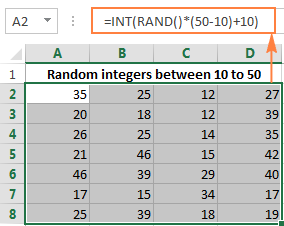## Excel RANDBETWEEN function - generate random integers in a specified range

RANDBETWEEN is another function provided by Excel for generating random numbers. It returns random integers in the range that you specify:

RANDBETWEEN(bottom, top)

Obviously, bottom is the lowest number and top is the highest number in the range of random numbers you want to get.

Like RAND, Excel's RANDBETWEEN is a volatile function and it returns a new random integer every time your spreadsheet recalculates.

For instance, to generate random integers between 10 and 50 (including 10 and 50), use the following RANDBETWEEN formula:

`=RANDBETWEEN(10, 50)`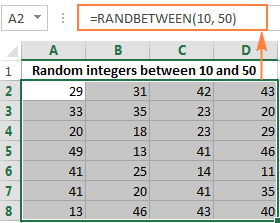The RANDBETWEEN function in Excel can create both positive and negative numbers. For example, to get a list of random integers from -10 to 10, enter the following formula in your worksheet:

`=RANDBETWEEN(-10, 10)`

The RANDBETWEEN function is available in Excel 365 - Excel 2007. In earlier versions, you can use the RAND formula demonstrated in Example 3 above.

Further on in this tutorial, you will find a few more formula examples demonstrating how to use the RANDBETWEEN function to generate random values other than integers.

Tip. In Excel 365 and Excel 2021, you can use the dynamic array RANDARRAY function to returns an array of random numbers between any two numbers that you specify.

## Create random numbers with specified decimal places

Though the RANDBEETWEEN function in Excel was designed to return random integers, you can force it to return random decimal numbers with as many decimal places as you want.

For instance, to get a list of numbers with one decimal place, you multiply the bottom and top values by 10, and then divide the returned value by 10:

RANDBETWEEN(bottom value * 10, top value * 10)/10

The following RANDBETWEEN formula returns random decimal numbers between 1 and 50:

`=RANDBETWEEN(1*10, 50*10)/10`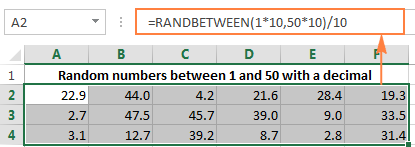In a similar manner, to generate random numbers between 1 and 50 with 2 decimal places, you multiply the RANDBETWEEN function's arguments by 100, and then divide the result by 100 as well:

`=RANDBETWEEN(1*100, 50*100) / 100`

## How to generate random dates in Excel

To return a list of random dates between the given two dates, use the RANDBETWEEN function in combination with DATEVALUE:

RANDBETWEEN(DATEVALUE(start date), DATEVALUE(end date))

For example, to get the list of dates between 1-Jun-2015 and 30-June-2015 inclusive, enter the following formula in your worksheet:

`=RANDBETWEEN(DATEVALUE("1-Jun-2015"),DATEVALUE("30-Jun-2015"))`

Alternatively, you can use the DATE function instead of DATEVALUE:

`=RANDBETWEEN(DATE(2015,6,1),DATEVALUE(2015,6,30))`

Remember to apply the date format to the cell(s) and you will get a list of random dates similar to this: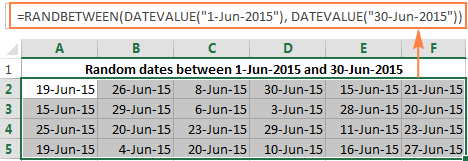For a number of advanced options such as generating random weekdays or weekends, check out Advanced Random Generator for dates.

## How to insert random times in Excel

Remembering that in the internal Excel system times are stored as decimals, you can use the standard Excel RAND function to insert random real numbers, and then simply apply the time format to the cells: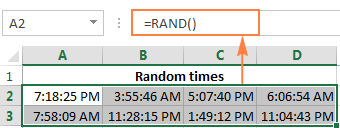To return random times according to your criteria, more specific random formulas are required, as demonstrated below.

### Formula 1. Generate random times in the specified range

To insert random times between any two times that you specify, use either the TIME or TIMEVALUE function in conjunction with Excel RAND:

TIME(start time)+RAND() * (TIME(start time) - TIME(end time))
TIMEVALUE(start time)+RAND() * (TIMEVALUE(start time) - TIMEVALUE(end time))

For example, to insert a random time between 6:00 AM and 5:30 PM, you can use either of the following formulas:

`=TIME(6,0,0) + RAND() * (TIME(17,30,0) - TIME(6,0,0))`

`=TIMEVALUE("6:00 AM") + RAND() * (TIMEVALUE("5:30 PM") - TIMEVALUE("6:00 AM"))`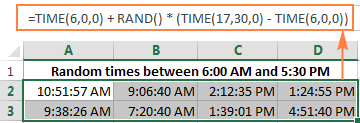### Formula 2. Generating random dates and times

To create a list of random dates and times, use a combinations of RANDBETWEEN and DATEVALUE functions:

RANDBETWEEN(DATEVALUE(start date), DATEVALUE(end date)) + RANDBETWEEN(TIMEVALUE(start time) * 10000, TIMEVALUE(end time) * 10000)/10000

Supposing you want to insert random dates between June 1, 2015 and June 30, 2015 with a time between 7:30 AM and 6:00 PM, the following formula will work a treat:

`=RANDBETWEEN(DATEVALUE("1-Jun-2015"), DATEVALUE("30-Jun-2015")) + RANDBETWEEN(TIMEVALUE("7:30 AM") * 10000, TIMEVALUE("6:00 PM") * 10000) / 10000`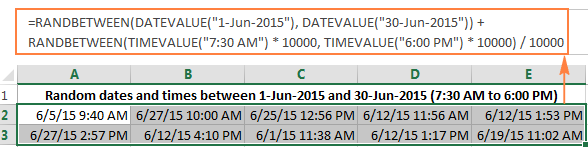You can also supply dates and times using the DATE and TIME functions, respectively:

`=RANDBETWEEN(DATE(2015,6,1), DATE(2015,6,30)) + RANDBETWEEN(TIME(7,30,0) * 10000, TIME(18,0,0) * 10000) / 10000`

## Generating random letters in Excel

To return a random letter, a combination of three different functions is required:

`=CHAR(RANDBETWEEN(CODE("A"),CODE("Z")))`

Where A is the first character and the Z is the last character in the range of letters you want to include (in alphabetical order).

In the above formula:

• CODE returns numeric ANSI codes for the specified letters.
• RANDBETWEEN takes the numbers returned by the CODE functions as the bottom and top values of the range.
• CHAR converts random ANSI codes returned by RANDBETWEEN to the corresponding letters.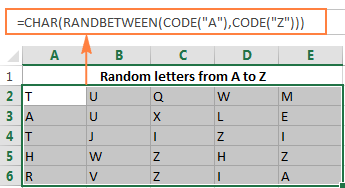Note. Since, the ANSI codes are different for UPPERCASE and lowercase characters, this formula is case-sensitive.

If someone remembers the ANSI Character Codes Chart by heart, nothing prevents you from supplying the codes directly to the RANDBETWEEN function.

For example, to get random UPPERCASE letters between A (ANSI code 65) and Z (ANSI code 90), you write:

`=CHAR(RANDBETWEEN(65, 90))`

To generate lowercase letters from a (ANSI code 97) to z (ANSI code 122), you use the following formula:

`=CHAR(RANDBETWEEN(97, 122))`

To insert a random special character, such as ! " # \$ % & ' ( ) * + , - . /, use the RANDBETWEEN function with the bottom parameter set to 33 (ANSI code for "!') and the top parameter set to 47 (ANSI code for "/").

`=CHAR(RANDBETWEEN(33,47))`

## Generating text strings and passwords in Excel

To create a random text string in Excel, you just have to concatenate several CHAR / RANDBEETWEEN functions.

For example, to generate a list of passwords consisting of 4 characters, you can use a formula similar to this:

`=RANDBETWEEN(0,9) & CHAR(RANDBETWEEN(65,90)) & CHAR(RANDBETWEEN(97, 122)) & CHAR(RANDBETWEEN(33,47))`

To make the formula more compact, I supplied the ANSI codes directly in the formula. The four functions return the following random values:

• `RANDBETWEEN(0,9)` - returns random numbers between 0 and 9.
• `CHAR(RANDBETWEEN(65,90))` - returns random UPPERCASE letters between A and Z.
• `CHAR(RANDBETWEEN(97, 122))` - returns random lowercase letters between a and z.
• `CHAR(RANDBETWEEN(33,47))` - returns random special characters.

The text strings generated with the above formula would be something like "4Np#" or "2Yu&".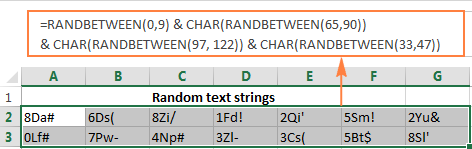A word of caution! If you use a similar formula to create random passwords, they won't be strong. Of course, there's nothing saying you can't generate longer text strings by chaining more CHAR / RANDBETWEEN functions. However, it's impossible to randomize the order or characters, i.e. the 1st function always returns a number, the 2nd function returns an uppercase letter and so on.

If you are looking for an advanced random password generator in Excel capable of producing text strings of any length and pattern, you may want to check out the capabilities of Advanced Random Generator for test strings.

Also, please keep in mind that the text strings generated with the above formula will change every time your worksheet recalculates. To ensure that your strings or passwords remain the same once they are created, you will have to stop the RANDBETWEEN function from updating the values, which leads us directly to the next section.

## How to prevent RAND and RANDBETWEEN from recalculating

If you want to get a permanent set of random numbers, dates or text strings that won't change every time the sheet is recalculated, use one of the following techniques:

1. To stop the RAND or RANDBETWEEN functions from recalculating in one cell, select that cell, switch to the formula bar and press F9 to replace the formula with its value.
2. To prevent an Excel random function from recalculating, use the Paste Special > Values feature. Select all the cells with the random formula, press Ctrl + C to copy them, then right click the selected range and click Paste Special > Values.

## How to generate unique random numbers in Excel

Neither of Excel's random functions can produce unique random values. If you want to create a list of random numbers without duplicates, perform these steps:

1. Use the RAND or RANDBETWEEN function to generate a list of random numbers. Create more values than you actually need because some will be duplicates to be deleted later.
2. Convert formulas to values as explained above.
3. Remove duplicate values by using either Excel's built-in tool or our advanced Duplicate Remover for Excel.

More solutions can be found in this tutorial: How to generate random numbers without duplicates.

## Advanced Random Number Generator for Excel

Now that you know how to use random functions in Excel, let me demonstrate you a faster, easier and formula-free way to create a list of random numbers, dates or text strings in your worksheets.

AbleBits Random Generator for Excel was designed as a more powerful and user-friendly alternative to Excel's RAND and RANDBETWEEN functions. It works with all versions of Microsoft Excel 2019, 2016, 2013, 2010, 2007 and 2003 equally well and addresses most of the quality and usability issues of the standard random functions.

#### AbleBits Random Number Generator algorithm

Before showing our Random Generator in action, let me provide a few key notes on its algorithm so that you know exactly what we are offering.

• AbleBits Random Number Generator for Excel is based on the Mersenne Twister algorithm, which is considered an industry standard for high-quality pseudo randomization.
• We use version MT19937 that produces a normally distributed sequence of 32-bit integers with a very long period of 2^19937 - 1, which is more than sufficient for all imaginable scenarios.
• Random numbers generated using this method are of a very high quality. The Random Number Generator has successfully passed multiple tests for statistical randomness, including the well-known NIST Statistical Test Suiteand Diehard tests and some of TestU01 Crush randomness tests.

Unlike Excel random functions, our Random Number Generator creates permanent random values that do not change when a spreadsheet recalculates.

As already noted, this advanced Random Number Generator for Excel offers a formula free (and consequently error-free :) way to create various random values such as:

• Random integers or decimal numbers, including unique numbers
• Random dates (workdays, weekends, or both, and optionally unique dates)
• Random text strings, including passwords of a given length and pattern, or by mask
• Random Boolean values of TRUE and FALSE
• Random selection from custom lists

And now, let's see the Random Number Generator in action, as promised.

### Generate random numbers in Excel

With AbleBits Random Number Generator, creating a list of random numbers is as easy as clicking the Generate button.

Generating unique random integers

All you have to do is select the range to be populated with random integers, set the bottom and top values and, optionally, check the Unique Values box.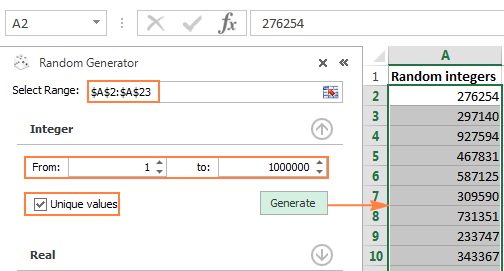Generating random real numbers (decimals)

In a similar manner, you can generate a series of random decimal numbers in the range that you specify.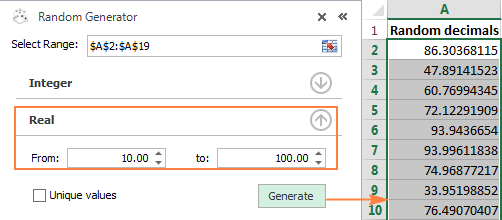### Create random dates in Excel

For dates, our Random Number Generator provides the following options:

• Generate random dates for a specific time period - you enter the bottom date in the From box and the top date in the To box.
• Include weekdays, weekends, or both.
• Generate unique dates.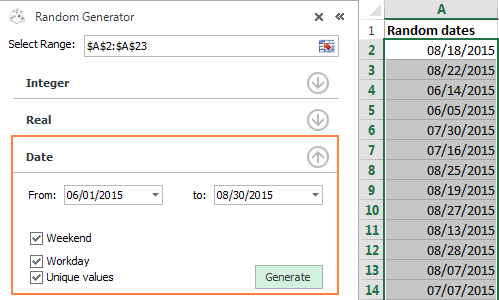### Generate random text strings and passwords

Apart from random numbers and dates, with this Random Generator you can easily create random alphanumeric strings with certain character sets. The maximum string length is 99 characters, which allows generating really strong passwords.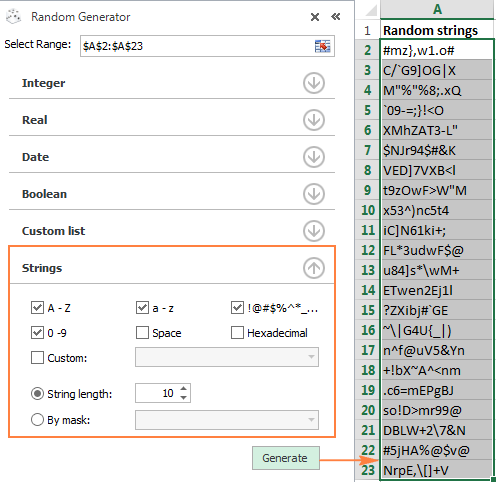A unique option provided by AbleBits Random Number Generator is creating random text strings by mask. This is a very useful feature for generating globally unique identifiers (GUID), zip codes, SKUs, and so on.

For example, to get a list of random GUIDs, you select the Hexadecimal character set and type ????????-????-????-???????????? in the Mask box, as demonstrated in the screenshot: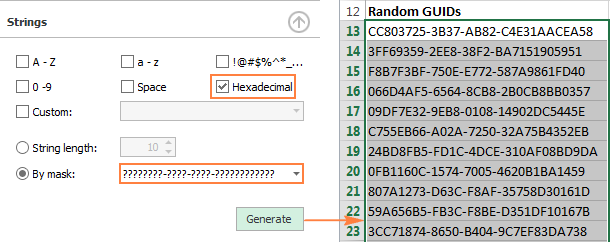If you are interested to give our Random Generator a try, you are most welcome to download it below as part of our Ultimate Suite for Excel.

Random formula examples (.xlsx file)
Ultimate Suite 14-day fully-functional version (.exe file)

## You may also be interested in

1. I have 4 numbers that need randomized. 25-50-75-100.
I need 7500 of the 25
3750 of the 50
1875 of the 75
and 1875 of the 100.

Is there a formula that can randomize specific values with specific quantities?

• Hi!
Looks like this is not possible with the standard Excel options. You can write all these 250 numbers on the worksheet, select that range of cells, and use tool Random Generator - Shuffle Cells. It is available as a part of our Ultimate Suite for Excel that you can install in a trial mode and check how it works for free.

• The closest I could get was with assigned percentages. 50%-25%-12.5%-12.5%. (F column)

I used =SUM(\$F\$2:F2) to get a Cumulative percent of 50%-75%-88%-100% (G column)

and then used this formula to get my result. It's not perfect but close enough for me to change a few to get my exact specific quantities.

=INDEX(E\$2:E\$5,COUNTIF(G\$2:G\$5,"<="&RAND())+1) (E column has the values of 25-50-75-100)

2. How can I apply the random formula to meet the following conditions. 1. a series of cells must equal a certain sum 2. They must be whole numbers.
Exampe: I want a column of blank cells to randomly add up to 20 every time I click generate? Thanks

3. I did random number between 3-18. I want to know that can I do the number which I want to start to random. How can I control these number unchange?

4. How can i have a random numbers those satisfies row and column totals in a contingency table.

• Hi!
Sorry, it's not quite clear what you are trying to achieve.

5. 205
227
152
153
199
150
174
237
231
?
?
?
how to find next random number by using previous random number between 150 to 250

• Hi!
If you tell me what the pattern is in these numbers, I will try to help write the formula.

6. Hi
We want to calculate random 8 values between 1-50, can you guide how to calculate?

• Hi!

7. i need this type of code

tsm 1923
tsm 9207

• Hi!
What do you want to calculate exactly? Your question is not entirely clear, please specify.

8. I have menus designed 2 per page that I want to print out with 35 different room numbers in sequence. This is out of my knowledge base and would love some help.

9. I need a solution for an eprime script. In my experiment I called images to appear randomly, but I dont want that a determined imaged will be selected more than one time. How can I randomize or pseudorandomize this task?

10. Expel
132
123
213
231
321
312
If I register a three digit number it is automatic
I need to change how I do this

• Hello!
I’m sorry but your task is not entirely clear to me. For me to be able to help you better, please describe your task in more detail. Please specify what you were trying to find, what formula you used and what problem or error occurred. Give an example of the source data and the expected result.
It’ll help me understand it better and find a solution for you. Thank you.

11. I want to generate a series of A’s and B’s but never more than 2 of A or B is it possible?

• Hello!
I’m sorry but your task is not entirely clear to me. For me to be able to help you better, please describe your task in more detail. Please specify what you were trying to find, what formula you used and what problem or error occurred. Give an example of the expected result.
It’ll help me understand it better and find a solution for you. Thank you.

12. Hola, quisiera saber cuál es la diferencia entre aleatorio y pseudoaleatorio?

• Hello Johan!

Random numbers are a sequence of numbers selected in a random way. Pseudo-random numbers are used instead of random ones. They are generated by a computer program using a predefined complex algorithm.
For an ordinary user they look like random, but still they can be predicted if you know that complex algorithm. Pseudo-random numbers are used in all computer programs.

13. What is the max capacity of RAND() in Excel - i.e. what number of i.i.d. random numbers one can safely simulate without running into autocorrelation of them (breaking statistical tests on i.i.d.)? Thanks

14. could you please any one help how to random using below string

Example :

SS
YS
TD
AR
KP
HH
ET
GD
JJ
KA
WC

15. Is there a way to use the RAND function with exceptions? I want to generate random numbers between 93 and 118 but they can not include 103 or 114. (Also each number can only appear a maximum of twice per row). Is there a better function to use?

16. Is it possible if
A=24
then B=random value less than 12
and C=random value less than 12
A=a+b

17. 1 180
2 180
3 360
4 180
5 180
6 360
7 180
8 180
9 360
10 540
11 180
12 180
13 180
14 720
15 540
16 360
17 180
18 180
19 360
20 540
21 360
22 180
23 360
24 180
25 720
26 900
27 1080
28 3600
29 9000
30 5400
31 2700
32 1800
33 1260
34 540
35 900
36 720
37 540
38 180
39 540
40 360
41 180
42 360
43 540
44 180
45 360
46 540
47 720
48 180
49 180
50 180

40860
want in cell two to select rendomly no so that there sum may be 18000 and 16200 like wise is this possible by any formula

18. how to generate random number (real) in a specified range that will have a Ppk value of at least 1.3

TIA

• Nincals:
Is something like this what you want?
=RANDBETWEEN(1.3*10,5*10)/10
The lowest value is 1.3, the highest value is 5 and the numbers will be decimal numbers. This idea is discussed in the above article.
You can format the cells as numbers to show one decimal place.

19. What is the best formula when rolling (many) dice:
a) The TRUNC(RAND) function
or
b) The INT(RAND) function
or
c) are they both suitable?

21. wow i like this app

23. Hi there, i want to generate random number between 2 number in decimal point form (between 0.1 and 0.6), but always got value of 1. Is there any possible way to make the random number generate between these 2 values?

24. I have a list of Latitude and Longitude values in excel, is there a way to build a script that will take the column of data and randomize the last 2 digits past the decimal? This would in effect jitter the points by a few meters, and keep points from all falling on top of each other on a map. Anyone know how to accomplish this?

25. I have a small VBA code setup to generate 4 random integers (using INT (RAND()*(10-1)+1) ) between 1 and 10 every time I push a button on the worksheet. What I noticed is that every time I open the spreadsheet it cycles through the same order of numbers. Meaning the first time I click the button I'll get the same 4 random numbers, the second time I push the button I'll get the same set of numbers I got the last time I opened the sheet and presssed it twice.

26. Hi, Would you please help me find the correct formula to generate a random number between 1500 and 4000 which ends in a zero (either round up or down, doesnt matter).
Kind regards

• Hi, Martin,

try the following formula:
=ROUND(RANDBETWEEN(1500,4000),-1)

You can find more about ROUND function here.

27. NOT TRUE;
There is no way to seed either Excel RAND or RANDBETWEEN function, which are rumored to be initialized from the computer's system time. Technically, a seed is the starting point for generating a sequence of random numbers. And every time an Excel random function is called, a new seed is used that returns a unique random sequence. In other words, when using the random number generator in Excel, you cannot get a repeatable sequence with the RAND or RANDBETWEEN function, nor with VBA, nor by any other means.

Use Rnd(-N) to generate a reproducible number.
Feed the previous results as a negative number to the succeeding Rnd calls, x1=RND(-Seed); x2=RND(-x1) etc.. to produce a repeatable sequence from a seed number.

• Hello!

Right, in Excel VBA you can initialize a random sequence using Rnd(-N), but as noted in the tutorial, it cannot be done with the regular worksheet functions RAND or RANDBETWEEN. Or course, you can create your own user-defined function that will use RND to generate reproducible numbers.

28. Hi, is there a possible way to combine the randbetween function with current day. In other words use a random number with current day to create an unique number using only the year and the day only. Thanks!

=text(today(),"DD ")&randbetween(100,9999)

29. Trying to randomize the 55- 2 digit combinations for 0-9. For example: 0,0 0,1 and so on through 9,9. 0,1 and 1,0 are considered the same combo. Gotta be easier than 55 slips of paper?? Can you help?

30. Very nice article. Note that from Excel 2010 onwards, Excel RAND() function uses the Mersenne Twister algorithm.

31. 3 cells wide 100 down that have my own numbers that don't change.

3 cells wide beside it and 100 down as well but random numbers between 1 and 20 "no decimals"

3 Cells wide 100 down as a match column that highlights any number of single pairs or tripples

Can you paste or send to my email please

32. I need random incremental decimal values in a range.

range
22.1234 to 79.1234

and from row 1 to row 300.

how to do it........?

33. how to generate the 100 random numbers equally
distributed between 23 and 87 using excel

34. Hello

i am working on a norm.inv(rand(),Mean,Std dev) using these mean and deviation values to generate my random number. however, the deviation may bring the value across 0, negative, which is not possible for my needs. is it possible to put a bounds (randbetween) while still asking it to use the mean and dev. to create the number?

35. Hi Svetlana, I have a requirement in excel when I simulate random variables in excel particular cell, if the cell value is within a specified range, I want to copy that value to a new cell each time it simulates, I want to retain the previous value to calculate the mean, Kindly help with the requirement of copying the simulated value to new cell I am running the simulation 10k times

36. When I say '5 rows', I mean as many times as posible for each number in the ninety numbers.

Urgently pls.

37. I want to generate a lotto chart of 1 - 90 in 5 unrepeated rows involving all numbers such that every winning result of 5 numbers must comform with at least one possible and correct row.

38. Hi

Do you know how to change the root of the generator? has excell a simple method to do that? thanks.

• Hello, Yasser,

If you mean the randomization algorithm, it cannot be changed. That's why we created Random Generator for Excel - to use a different reliable algorithm.

39. Is the specific method of generating RAND() in Excel known, or is it a black box? In other words, how random is RAND()?

• Hi TG,

The RAND function generates pseudo-random numbers from the Uniform distribution, aka rectangular distribution, where there is equal probability for all values that a random variable can take on.

40. I have several days extending for over 4 years
I need to generate one randon time for each date for the next 4 years

41. I have a date field and a time field in my excel table
For each day there are multiple times
20-1-2009 6:00
20-1-2009 7:00
20-1-2009 8:00

so on till
20-1-2009 24:00
from each day I need to generate one randon time from all times I have.

Can you help